Impact Factor 2.705 | CiteScore 2.2
More on impact ›

# Frontiers in Materials## Original Research ARTICLE

Front. Mater., 11 April 2019 | https://doi.org/10.3389/fmats.2019.00050

# Analysis of Low Frequency Acoustic Stop Bands in Cubic Arrays of Thick Spherical Shells With HolesGuillaume Dupont1,Alexander Movchan2,Stefan Enoch3 and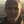Sébastien Guenneau3*
• 1Aix Marseille Univ, CNRS, Centrale Marseille, IRPHE, Marseille, France
• 2Department of Mathematical Sciences, The University of Liverpool, Liverpool, United Kingdom
• 3Aix Marseille Univ, CNRS, Centrale Marseille, Institut Fresnel, Marseille, France

We analyse the propagation of airborne pressure waves through a three-dimensional array of rigid coated spheres (shells) in air. When we dig a channel terminated by an air cavity in each rigid shell we observe the appearance of a low frequency stop band. Each shell with a hole acts as a Helmholtz resonator supporting a low frequency localized mode. Isofrequency surfaces and contours reveal the strong anisotropy of the periodic structure at the edge of the stop band. A simple mechanical model of springs and masses allows for asymptotic estimates of the low frequency stop band for elongated channels. Increasing the radius of an air channel shifts up the position, and enlarges, the low frequency stop band. Adding holes in shells also shifts up the frequency of the stop band, and embedded shells lead to additional stop bands. Localization effect induced by a large defect in a periodic macrocell of Helmholtz resonators is finally investigated.

## 1. Introduction: Acoustic Metamaterials

In the tracks of photonic crystals, phononic crystals (Dowling, 2008) have provided a fillip for research in acoustic stop band structures (Kushwaha et al., 1993; Kafesaki and Economou, 1999) within which light or sound is prohibited to propagate due to multiple scattering between periodically spaced inclusions. In 2000, Liu et al. provided the first numerical and experimental evidence of frequency dispersive elastic parameters of locally resonant structures for elastic waves in three-dimensional arrays of thin coated spheres (Liu et al., 2000): The effective parameters were shown to turn negative where low frequency stop bands occur. This important work paved the way toward acoustic analogs of electromagnetic meta-materials, such as fluid-solid composites for the control of pressure waves (Auriault and Bonnet, 1985; Auriault, 1994; Liu et al., 2000; Goffaux et al., 2002; Ho et al., 2003; Elford et al., 2004; Hirsekorn et al., 2004; Li and Chan, 2004; Movchan and Guenneau, 2004; Fang et al., 2006; Mei et al., 2006; Guenneau et al., 2007b; Hou et al., 2007; Wang et al., 2008; Chalmers et al., 2009; Norris, 2009; Lemoult et al., 2011; Auriault and Boutin, 2012; Boutin, 2013; Boutin and Becot, 2015). Using asymptotic methods for fields in multi-structures (Kozlov et al., 1999; Movchan et al., 2002), it has been proposed to use arrays of cylinders with a split ring cross section as building blocks for two-dimensional localized resonant acoustic structures displaying negative refraction (Movchan and Guenneau, 2004; Guenneau et al., 2007a), based on analogies with split ring resonators (SRRs), introduced by Pendry in the context of electromagnetic waves almost 20 years ago (Pendry et al., 1999). Magnetic activity of metamaterials occurs near resonances of SRRs, which was essential for instance in the design of the first electromagnetic cloak (Schurig et al., 2006), and similar designs have been proposed for acoustic cloaks (Craster et al., 2013).

In a series of articles, the research group of Auriault developed asymptotic models of locally resonant structures (Auriault and Bonnet, 1985; Auriault, 1994; Auriault and Boutin, 2012), some of which predate the birth of acoustic metamaterials with the seminal work of the research group of Ping Shen (Liu et al., 2000). Low frequency stop bands in arrays of 2D Helmholtz resonators with elongated necks have been studied in (Movchan and Guenneau, 2004; Guenneau et al., 2007a) as mentioned above, with further predictions and measurements of sound transmission in elastic shells in air in Krynkin et al. (2010). However, 3D Helmholtz resonators with elongated neck position inside the cavity and periodically distributed in a fluid have been introduced in (Boutin, 2013; Boutin and Becot, 2015). Therein, Boutin and coauthors have shown that the macroscopic fields (pressure P and velocity V) satisfy the following equation of mass conservation and generalized Darcy Law:

where K is the effective bulk modulus (including thermal effects) of the matrix, ω the resonant frequency, i2 = −1, Φ and T are the porosity and dynamic permeability tensor (including viscosity effects) when considering the resonators as perfectly rigid spheres, η is the viscosity of the fluid-matrix, ∣Y∣ is the volume of the 3D unit cell Y and Q is the flux emitted by the resonator into the fluid matrix in response to the pressure acting on it. The case of the Helmholtz resonator with a single elongated neck has been not only derived, but also experimentally tested, in Boutin (2013) and Boutin and Becot (2015). And the fluid need not be inviscid.

There is a vast amount of literature on the role of the neck in such Helmholtz resonators (Groeneweg, 1969; Alster, 1972; Orris et al., 1974; Gaunaurd and Uberall, 1982; Lim et al., 1990; Hinders et al., 1995; Selamet and Dickey, 1995; Mead, 1996; Aberg et al., 1997; Selamet et al., 1997; Chen et al., 1998; Baird et al., 1999; Seo et al., 2005; Ivansson, 2006; Duan et al., 2007; Zhao et al., 2007; Zhou et al., 2010; Wang and Mak, 2012; Li et al., 2013). Recently, periodic structures consisting of SRRs have been revisited thanks to refined homogenization techniques, which allow to rigorously link the geometrical parameters to frequency dependent effective parameters (Mercier et al., 2017). Analogs of SRRs have been proposed for in-plane elastic waves (Guenneau et al., 2007b), that allows for bending and rotational vibrations having lower resonant frequencies than compressional vibrations, and thus make good candidates for building blocks of mechanical metamaterials, which might make possible some mechanical cloak, see Kadic et al. (2013) for a review of metamaterials beyond electromagnetics. Milton, Briane and Willis provided a thorough mathematical frame for cloaking for certain types of elastodynamic waves in structural mechanics, in the framework of modified Willis equations (Milton et al., 2006). On the other hand, coupled in-plane pressure and shear waves were numerically shown to be detoured around a finite size obstacle by a specially designed cloak with an anisotropic heterogeneous elasticity tensor without the minor symmetries (Brun et al., 2009) without resorting to Willis media. Nonetheless, SRRs like in Guenneau et al. (2007b) make an interesting candidate to achieve such Cosserat-type metamaterials. Actually, a similar type of resonant elastic structure already led to a negatively refracting medium (Zhu et al., 2014), where the elastic chirality (which is a hallmark of a Cosserat medium) was put forward in conclusive experiments. The need for mechanical metamaterials with elasticity tensor without the minor symmetry motivates the extension of homgenization results of Mercier et al. (2017) to the Navier system. Importantly, the acoustic wave equation is invariant under coordinate changes (Norris, 2008, 2009), so acoustic metamaterials via geometric transform can thus achieve enhanced control of pressure waves via a simpler route. A simple example of a periodic system is a periodic lattice, whose dynamic response is well defined by Green's functions. Dynamic Green's functions in periodic lattices and their asymptotics were analyzed in Movchan and Slepyan (2014) and Vanel et al. (2016). Localization of electromagnetic waves within a two-dimensional grating of spheres embedded in the three-dimensional space and the high-frequency homogenisation approximations were studied in Maling et al. (2017).

A downfall of SRRs is that they do not allow for doubly negative acoustic parameters, so one would need a second type of inclusion within the periodic cell to achieve a goal of a negative refractive index in order to design a super lens like Pendry proposed in his seminal paper (Pendry, 2000). In 2004, Li and Chan proposed a design of negative acoustic metamaterial based on a multiple scattering theory approach (Li and Chan, 2004), which has been revisited recently by applied mathematicians using homogenization theory for bubbly fluids (Ammari et al., 2017a,b) with hybridization of Minnaert resonances (Minnaert, 1933). This is somewhat related to homogenization of high-contrast periodic structures (Figotin and Kuchment, 1998). Potential applications of bubbly media in super lensing and total absorption have been experimentally shown (Lanoy et al., 2015; Leroy et al., 2015). Such soft metamaterials (Brunet et al., 2015) are currently investigated by many groupings in the world, but they might not always be compatible with industrial processes.

Although SRRs might not achieve double negative acoustic parameters, they seem to be quite straightforward to engineer for ultrasonic waves. Fang et al. experimentally demonstrated a dynamic effective negative stiffness in a chain of air filled Helmholtz's resonators for ultrasonic waves (Fang et al., 2006). Moreover, it has been also shown using asymptotic techniques that surface water waves propagating within an array of fluid filled SRRs display a negative effective density (Farhat et al., 2009). A focussing effect through a finite array of such resonators was numerically achieved, with a resolution of a third of the wavelength. The negative effective density, reinterpreted as a negative effective gravity, has been further confirmed theoretically and experimentally (Hu et al., 2011, 2017). Fluid filled SRRs have been experimentally tested in a 17 meter long water channel for filtering effects with a dike using grooved vertical cylinders (Dupont et al., 2017). Similar filtering effects have been experimentally demonstrated for sound waves interacting with a doubly periodic array of Helmholtz's resonators shaped as soda cans (Lemoult et al., 2011). However, we note that in that case, there is no neck, just a hole, as soda cans are thin shells. In the present paper, we would like to revisit the concepts of SRRs in the case of pressure waves propagating in a three-dimensional array of 3D Helmholtz resonators with elongated necks. In order to simplify the mathematical setup, we shall consider airborne acoustic waves, in which case rigid (Neumann type) boundary conditions can be considered on resonant elements. Compared to problems of linear elasticity, the present study does not deal with dynamic degeneracies at low frequencies, which may occur for certain type of geometries of elastic systems, resulting in a group of very small eigenvalues being separated from the remaining spectrum.

## 2. Motivation: Spectral properties of a periodic array of rigid spheres

Let us start with an illustrative numerical result for a spectral problem for the Helmholtz operator within a periodic cubic array of rigid spheres: the unknown is a pressure wave field, here sound in air (wave speed c = 340m.s−1). Neumann boundary conditions are prescribed on the contour of each defect and standard Floquet-Bloch conditions are set on an elementary cell of the periodic structure. The finite element formulation was implemented in the COMSOL Multiphysics Package to compute the eigenvalues and to generate the corresponding eigenfields. We present in Figure 1 the periodic structure we want to study and in Figure 2 the corresponding dispersion diagram for eigenfrequencies ω (in unit of rad.s−1) as a function of the Floquet-Bloch parameter k (in unit of m−1): along the horizontal axis we have the values of modulus of k, where k stands for the position vector of a point on the contour ΓXMU within the irreducible Brillouin zone. We note the absence of bandgaps with the presence of rigid spheres. This lack of intervals of forbidden frequencies motivates the present study: how can one create a stop band without further increasing the size of the rigid spheres?

FIGURE 1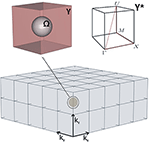Figure 1. Three-dimensionnal phononic crystal, periodic cell Y with a rigid sphere Ω in physical space and irreducible Brillouin zone ΓXMU of the periodic cell Y* in reciprocal space with the three components of the Floquet-Bloch vector k = (kx, ky, kz).

FIGURE 2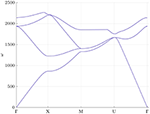Figure 2. Dispersion diagram for a periodic array (pitch d = 1 m) of spherical rigid inclusions (R = 0.4 m) representing the frequency ω (rad.s−1) of pressure waves in air, vs. the wavenumber |k| (m−1), projection of the Bloch vector k along the edges of ΓXMU.

## 3. Setup of the spectral problem: The continuum model

Let us first recall the finite element set-up. We consider the Helmholtz equation:

where ρ (kg.m−3), K (Pa) are the density and bulk modulus of the medium and ω (rad.s−1) is the angular wave frequency of the pressure field p.

Due to the periodicity of the lattice, we look for solutions of (2) in terms of Floquet-Bloch waves (Floquet, 1883; Bloch, 1928; Bensoussan et al., 1978; Brillouin, 1978; Wilcox, 1978; Kittel, 1986). So, for a cubic array of unit cells Y,

where kx, ky and kz are components of the Bloch vector k within the Brillouin zone Y* = [0, π]3 (Joannopoulos et al., 1995; Gazalet et al., 2013).

The implementation in the finite element package is fairly straightforward (Hladky-Hennion et al., 1991; Nicolet et al., 2004). We first multiply equation (2) by a smooth test function v and using integration by parts, we obtain the so-called weak form of the time-harmonic acoustic equation

where ∂f/∂n = ∇f·n with n the unit outward normal to the boundary ∂Y of Y, and ds the infinitesimal surface element on ∂Y.

We note that the weak formulation holds for heterogeneous fluids as ρ and K can be spatially varying. In particular, this model works for domains such as a homogeneous fluid filled with a periodic array of rigid obstacles. For the finite element implementation, (4) is discretised using test functions taking values on nodes of a tetrahedral mesh of the basic cell (first order tetrahedral elements), see e.g., (Nicolet et al., 2004) for further details. From (4), we note that setting rigid boundary conditions on an inclusion amounts to assuming Neumann (natural) homogeneous data, whereas transmission conditions at the interface between various fluid phases mean that the quantity ρ−1p/∂n is preserved across the interface. We note that in the case of airborne pressure waves, the contrast in density between air and inclusions made of metal or even polymer is sufficiently large to assume Neumann data, but this simplification does not hold if we replace air by water.

Let us now consider a periodic array of defects Ω1, …, ΩN embedded in an elementary cell Y = ]0;1[3. Let p(x, y, z) satisfy the Helmholtz equation in $Y\{\bigcup }_{j=1}^{N}\overline{{\Omega }_{j}}$. We also assume that p satisfies Neumann boundary conditions on the contours of defects, where n denotes the unit outward normal to the boundary ∂Ωj of a defect Ωj:

We would like to consider a particular case when the defects Ω1, …, ΩN are spherical shells with thin air channels connecting an air-filled interior cavity to the exterior surrounding air. These defects can be modeled as multistructures (Kozlov et al., 1999) in the following way,

where a(N) and b(N) are given constants and ${\Pi }_{\epsilon \left(N\right)}^{\left(j\right)}$ is the thin channel.

## 4. Asymptotic Approximation: A Discrete Spring-Mass Model

In this section, we derive an asymptotic approximation of the field in thin channels ${\Pi }_{\epsilon }^{\left(j\right)}$, see Figure 3,

where lj is the length of the jth bridge, εhj(t) the radius of its varying cross-section Dε (parametrized by a positive real t). Here, ε is a small positive non-dimensionnal parameter. To derive the asymptotic expansions, we introduce the scaled variables ξ = (y/ε, z/ε).

FIGURE 3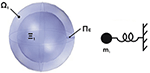Figure 3. Geometry of the inclusions and the Helmholtz oscillator consisting of one spring connected to a mass at one end and fixed at the other end.

Without loss of generality, and for the sake of simplicity, we drop the superscript j. In Πε, the time-harmonic wave equation takes the rescaled form

with the Neumann boundary conditions

The field p is approximated in the form

Hence, p(0) = p(0)(x) (it is ξ−independent). Assuming that p(0) is given, we derive that the function p(1) satisfies the following model problem on the scaled cross-section of Πε

The condition of solvability for the problem has the form:

Hence, we have shown that to the leading order we can approximate the field p in the thin channel Πε by the function p(0) which satisfies the Helmholtz's equation in one-space dimension. We now assume that the field is periodic over the cell since it is localized. This shows that the average of the eigenfield over the macro-cell vanishes. Indeed, let χ1 denote the value of the field in the large body Σ of the multi-structure Ω and let χ2 (which we normalize to 1) denote the value of the field within the complementary area of the macro-cell Y \ Ω excluding the thin channels. Taking v = 1 in (4), we deduce that

This shows that the average of the field p over Y vanishes, hence by neglecting the small volume of the thin channels, we obtain

where measΣ and measY \ Ω denote, respectively the volumes of Σ and Y \ Ω.

We now consider two cases. The first one is the study of an array of simple spherical shells with either one or six thin channels, and the other one is the study of an array of double spherical shells with one thin channel in each shell. Since we have q thin channels, we have q separate eigensolutions Vj, (j = 1, …, q), corresponding to the vibrations of thin domains ${\Pi }_{\epsilon }^{\left(j\right)}$

We note that Vj(0) is equal to a non-zero constant and also that Vj(lj) = χ1. Next, we need to take into account that some boundary layers occur at the end regions of thin ligaments. These boundary layers are characterized by exponential decay when the boundary conditions for the functions V0 (the leading term in the asymptotic expansion of the thin bridge solutions) are chosen in a specific way. In our case, integrating (2) over $\Sigma \cup {\Pi }_{\epsilon }^{\left(1\right)}\cup \cdots \cup {\Pi }_{\epsilon }^{\left(q\right)}$ and applying the divergence (or Gauss) theorem, we obtain to order O(ε)

where

All the channels are connected to Ξ, hence, V1(l1) = … = Vq(lq) = V. We note that the boundary layer condition (18) can be interpreted as Newton's second law.

The solution of the problem (16)−(18) (that one can interpret in terms of a spring-mass model like in Figure 3) has the form

where $c=\sqrt{K/\rho }$ and the frequency ω is given as the solution of the following equation:

where we invoked (18). Looking at a first low frequency, we deduce an explicit asymptotic approximation

This estimate actually holds for the frequency ω2 of the upper edge of the phononic band gap. We note that if we take V(0) = 0 instead of V(0) = meas(Ξ)/meas(Y \ Ω), we estimate the frequency of the lower-edge of the phononic band gap. We also notice that the boundary layer condition (18) is only valid at order 0(ε), so it needs to be refined if one wants to improve the frequency estimate in (22).

### 4.1. Eigenfrequency Estimate in the Case of a Single Spherical Shell With one or six Thin Channels

We report in Figures 4, 5 finite element computations for a periodic cell of sidelength d = 1 m with a simple spherical shell with one thin channel. We then proceed with the same shell with six thin channels. The geometry of the elementary cell and associated spring-mass model are given in Figure 6 and finite element computations are shown in Figures 7, 8. The interior and exterior radii of the shell are respectively 0.3 and 0.4 m, the thin channels have the same length 0.1m and radii 0.01 m. Therefore, the frequency estimates are (in rad.s−1):

for one thin channel, which are in good agreement with the finite element values

for one thin channel, and

for six thin channels, which are in good agreement with the finite element values

for six thin channels.

FIGURE 4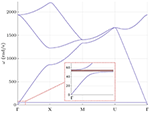Figure 4. Dispersion diagram for a periodic array (pitch d = 1m) of spherical rigid shells (inner radius 0.3 m and outer radius 0.4 m) with one thin channel (length 0.1 m and radius 0.01 m) representing the frequency ω (rad.s−1) of pressure waves in air vs. the wavenumber k = |k| (m−1), projection of the Bloch vector k along the edges of the irreducible Brillouin zone ΓXMU shown in Figure 1. We note the appearance of a frequency stop band for ω ∈ [51.4250, 55.2638] rad.s−1.

FIGURE 5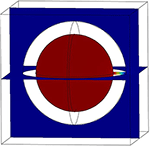Figure 5. The eigenfunction corresponding to the eigenfrequency ${\omega }_{1}^{*}=51.4250$ rad.s−1 for one thin channel. Blue color corresponds to nearly vanishing amplitude of the eigenmode u, while red color corresponds to it maximum value. The pressure field p is constant inside the inner cavity and outside the shell, but it varies rapidly inside the thin channel: it is a localized eigenmode responsible for the stop band in Figure 4, which is well approximated by a spring mass model.

FIGURE 6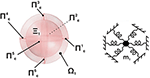Figure 6. Geometry of the inclusions and the Helmholtz oscillator consisting of six springs connected to a mass at one end and fixed at the other end.

FIGURE 7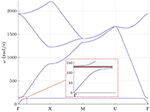Figure 7. Dispersion diagram for a periodic array (pitch d = 1 m) of spherical rigid shells (inner radius R = 0.3 m and outer radius R = 0.4 m) with six thin channels (length 0.1 m and radius 0.01m) representing the frequency ω (rad.s−1) of pressure waves in air vs. the wavenumber |k| (m−1), projection of the Bloch vector k along the edges of the irreducible Brillouin zone ΓXMU. We note the appearance of a frequency stop band for ω ∈ [125.6586, 134.8155] rad.s−1 which is wider and at higher frequencies than the stop band in Figure 4: the more identical thin channels, the higher the resonant frequency of the localized mode.

FIGURE 8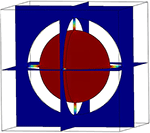Figure 8. The eigenfunction corresponding to the eigenfrequency ${\omega }_{1}^{*}=125.6586$ rad.s−1 for six thin channels responsible for the stop band in Figure 7. This frequency is well approximated by the spring mass discrete model which provides us with the frequency estimate ω1 = 127.6447 rad.s−1.

This demonstrates that the discrete model provides accurate estimates for the lower and upper edges of the ultra-low frequency stop band. This is therefore a useful tool which can be used in the design of acoustic metamaterials. Importantly, we note that the spring-mass counterpart of the shell with 6 holes, is like in Figure 6, which corresponds to an LC electrical circuit with one capacitance C and six inductances L in series corresponding to the mass and springs, respectively, see e.g., Guenneau et al. (2007a) for an analysis of two-dimensional acoustic metamaterials.

### 4.2. Dependence of low Frequency Stop Band on Radius of Thin Channel

Before we move to more complex geometries, we would like to investigate the case of Helmholtz resonators with thicker channels, that would prevent viscous effects for pressure waves entering the thin channels in manufactured prototypes. We note that there is an obvious limitation of the asymptotic model. Indeed, the accuracy of the eigenfrequency estimate relies heavily on the smallness of the parameter ϵ, which essentially requires elongated channels (in other terms ligaments or necks). We have checked the lack of robustness of the asymptotic estimates with respect to the elongation of channels, when we changed the radius of the thin channel for a Helmholtz resonator with a single channel like in Figure 3. We therefore focus on finite element results which are shown in Table 1, and one can see that although the low frequency stop band is preserved for increasing values of the radius, its position is shifted up and it is enlarged. These results are good news for forthcoming experiments.

TABLE 1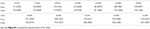Table 1. Values of the extrema of the gap with respect to the radius of the thin channel, for the Helmholtz resonator like in Figure 3.

FIGURE 9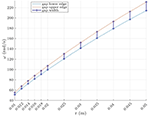Figure 9. Modification of the gap width and position with respect to the radius of the thin channel of the Helmholtz resonator with a single shell like in Figure 3. The eigenfrequency estimates ω1~52.1107 and ω2~54.0796 compare well with finite element results ${\omega }_{1}^{*}~51.4250$ and ${\omega }_{2}^{*}~55.2638$ for r = 0.01m, see Table 1. Note the non linear scale for r.

### 4.3. Eigenfrequency Estimate in the Case of a Double Spherical Shell With One Thin Channel

Let us now consider the case of and LC circuit with more than one capacitance. The simplest model of interest is that of an LC circuit with two capacitances and two inductors. This corresponds to a mechanical model like in Figure 10.

FIGURE 10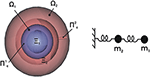Figure 10. Geometry of the inclusions and the Helmholtz oscillator consisting of two masses connected by a spring, with one of them connected to a fixed domain.

In the numerical example we now have ${\epsilon }^{2}{h}_{2}^{2}=3.14×1{0}^{-4}$m2, ${\epsilon }^{2}{h}_{1}^{2}=7.85×1{0}^{-5}$m2, l2 = 0.1m, l1 = 0.05m, and the masses (in kilogram)

where ρ is the density of air (~1.225kg.m−3), V1 and V2 the volumes air occupies in Ξ1 and Ξ2, r1 is the interior radius for the domain Ξ1 and a2, b2 are respectively the interior and exterior radii for the domain Ξ2. In our case, r1 = 0.1m, a2 = 0.15m and b2 = 0.2m. The formula (22) gives the following values for the first eigenfrequencies (in rad.s−1) of the multistructures Πε(1)⋃Ξ(1) and Πε(2)⋃Ξ(2):

The corresponding angular frequencies (in rad.s−1) associated with the standing waves in the periodic structure were obtained numerically, and from the band diagram in Figure 11 they are

FIGURE 11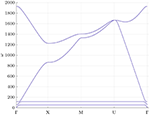Figure 11. Dispersion diagram for a periodic array (pitch d = 1m) of double spherical rigid shells (radius of spheres from inner to outer are 0.1, 0.15, 0.3, and 0.4 m) with one thin channel in each shell (respectively of lengths 0.05 and 0.1 m and radii 0.005 and 0.01 m) representing the frequency ω (rad.s−1) of pressure waves in air vs. the wavenumber k = |k| (m−1), projection of the Bloch vector k along the edges of the irreducible ΓXMU. We note the appearance of two frequency stop bands for ω ∈ [55.2128, 58.5863] rad.s−1 and ω ∈ [118.9750, 119.0959] rad.s−1.

Formula (22) leads to frequency estimates in (28) that do not capture the eigenfrequency ${\omega }_{1}^{*}$, that corresponds to the localized eigenmode shown in the left part of Figure 12, but we observe a good agreement between frequency estimate ω1 and ${\omega }_{2}^{*}$, associated with the eigenmode shown in right part of Figure 12. The estimate for the eigenfrequency ${\omega }_{1}^{*}$ can be found if the domain Πε(2)⋃Ξ(2) is replaced by the domain Πε(2)⋃Ω(2)⋃Πε(1)⋃Ω(1). In this case, the eigenfrequency ${\omega }_{1}^{*}$ is approximated by the first positive eigenvalue of the problem

where V1(x), V2(x) are the eigenfunctions defined on (0, l1) and (0, l2), respectively, and the masses m1, m2 are defined by (in kilogram)

FIGURE 12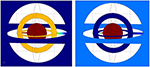Figure 12. Eigenfunctions for embedded resonators corresponding to the eigenfrequency ${\omega }_{1}^{*}=55.2128$ rad.s−1 (left panel) and corresponding to the eigenfrequency ${\omega }_{2}^{*}=118.975$ rad.s−1 (right panel). In left panel both canals vibrates, while in right panel only the inner canal does. The second eigenfrequency ${\omega }_{2}^{*}=118.975$ rad.s−1 is reasonably well approximated by the first eigenfrequency of the spring mass model which gives and ω1 = 124.2641 rad.s−1 and ω2 = 208.4109 rad.s−1.

Taking into account that ω0 = O(ε), we deduce that it can be approximated as the first positive solution of the following algebraic equation:

so that ω0~82.76057 rad.s−1, which provides a reasonably accurate approximation of ω1* = 55.2128 rad.s−1. However, a further refinement of this asymptotic estimate would require adding higher order corrections and thus solving boundary layer type problems as discussed in Kozlov et al. (1999), and this falls beyond the scope of the present paper.

## 5. Isofrequency Surfaces, Dynamic Effective Anisotropy and Localized Modes

Thus far, we have only analyzed dispersion curves, but it has been known for over 80 years that one needs to be extra careful regarding analysis of say electronic band structures (Bouckaert et al., 1936), as there are examples showing that stop band edges might be reached strictly within the Brillouin zone, and so it is not enough to describe its edges to characterize the band spectrum (Harrison et al., 2007). We would like to investigate dynamic effective anisotropy effects near the edge of the low frequency stop band, and localization effects induced by local resonators such as in Movchan et al. (2006) ,Bigoni et al. (2013) ,Craster et al. (2013), and Llewellyn Smith and Davis (2010) for a frequency on the acoustic band.

Here, we provide representation for isofrequencies near the lower edge of the first stop band for the case of a Helmholtz resonator with one thin channel as in Figure 4. However, we observed very similar features in all other cases. We first note in Figure 13 that the distortion of the isofrequency surfaces increases when we move toward the lower edge of the stop band. A small change in the frequency leads to a dramatic change in the isofrequency surface. Besides, the observation of isofrequency (or slowness) contours in Figure 13 confirms that anisotropy increases near the edge of the stop band as contours become more and more elongated. Researchers in photonics used vanishing group velocity near stop band edges to achieve self-guiding of light in 2D photonic crystals in the past 20 years (Kosaka et al., 1999; Witzens et al., 2002; Chigrin et al., 2003). Such an effect is known in the applied mathematics and waves literature as a dynamic effective anisotropy (Slepyan et al., 1987; Ayzenberg-Stepanenko and Slepyan, 2008; Craster et al., 2009, 2010; Colquitt et al., 2012; Schnitzer, 2017) : for an isotropic medium, the isofrequency surface is spherical, and the corresponding isofrequency contours are circular. On the edge of the stop band, the extreme elongation of isofrequency contours reveals an extreme dynamic anisotropy. We note that some dynamic effective anisotropy of 3D dynamic lattices displays similar isofrquency surfaces to ours near stop band edges (Vanel et al., 2016). This could be used to achieve as aforementioned highly directive phenomena, in a way similar to what was demonstrated experimentally for transverse electromagnetic waves (Ceresoli et al., 2015). Another striking effect is that of wave localization, when one creates one or more defects in a periodic structure. Usually, this is achieved in high frequency stop bands, when the wave wavelength is on the same order as the periodicity. Here, the wave localization is achieved either at resonances of resonators, see upper panel in Figure 14, or as shown in the lower panel of the same figure, at a frequency ${\omega }_{1}^{*}=559.2042$ rad.s−1 located in a part of the acoustic band sandwiched between the low frequency stop band and another region of vanishing group velocity near the X symmetry point in Figure 4, so this has the additional feature of being below the Bragg frequency regime. We note that from Figure 14, this localized mode can be approximated as a monopole given by the first derivative of the spherical Bessel function of the first kind jnr/c), where r is the radial position. The lowest root corresponding to the lowest frequency of the cavity is the frequency given by the root of the transcendental equation (simply derived by separation of variables in Helmholtz's equation (2) written in spherical coordinates and assuming that ∂p/∂n = 0 on the boundary of the defect) :

Bearing in mind that the first root of ${j}_{1}^{\prime }$ is 2.0816 and taking a = 1.25m as the radius of a dashed circle within which the defect mode seems to be mostly confined in Figure 14, (38) leads to the frequency estimate ω1 = 566.19 rad.s−1, which is in good agreement with the finite element computation ${\omega }_{1}^{*}=559.2042$ rad.s−1. This method of the effective sphere is the analogous concept to effective disk in doubly periodic structures with defects (Movchan et al., 2007).

FIGURE 13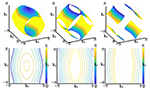Figure 13. Representative isofrequency surfaces kz(kx, ky) in the first Brillouin zone (upper panel) and associated isofrequency contours in the plane (kx, ky) for kz ∈ (0, π) (lower panel) at frequency ω = 51.42 rad.s−1 (left panel), ω = 51.4215 rad.s−1 (middle panel) and ω = 51.423 rad.s−1 (right panel); The surfaces and contours flatten when the frequency moves toward the lower edge of the stop band in Figure 4. and thus dynamic effective anisotropy increases.

FIGURE 14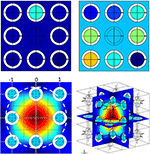Figure 14. Representative eigenfunctions for a macrocell of 26 resonators as in Figures 4, 5 with a defect (air instead of resonator) in the middle. Upper panel: Eigenfunctions corresponding to an eigenfrequency ${\omega }_{0}^{*}=52.3545$ rad.s−1 in the ultra-low frequency stop band of Figure 4. Lower panel: Eigenfunction corresponding to an eigenfrequency ${\omega }_{1}^{*}=559.2042$ rad.s−1 above the low frequency stop band of Figure 4, which is approximated by the first root of (38) i.e., the fundamental resonance of an effective spherical cavity (marked by dashed circle) of radius a = 1.25 m.

## 6. Conclusion

In this paper, we have seen that it is possible to sculpt the Bloch spectrum of three-dimensional phononic crystals almost ad libitum simply by digging some holes and adding cavities in rigid spheres periodically arranged along a cubic lattice. One of the main achievements of our numerical study is the appearance of ultra-low frequency stop bands at frequencies predicted quantitalively by an asymptotic model, that can be viewed as a 3D counterpart of (Movchan and Guenneau, 2004). We also conducted some elementary shape optimization (by varying the size, diameter and number of channels in a rigid sphere of constant radius) in order to enhance the control of the location and the number of low frequency stop bands, thanks to our asymptotic estimates. Our asymptotic results could be further used for a homogenization study in the spirit of the 2D analysis conducted in Mercier et al. (2017) to address the effective parameters of our acoustic system. However, the acoustic metamaterial we have analyzed would allow only frequency dependent effective bulk modulus, and in order to achieve a frequency dependent effective density and thus double negative acoustic parameters near resonances, one needs to introduce a second type of resonator in the periodic cell. Therefore, achieving a design of an acoustic superlens through negative refraction requires a more complex design. However, a cubic array of rigid spheres does not support any complete stop band, even in the densely packed configuration, so the acoustic metamaterial which we studied has markedly different filtering properties compared with phononic crystals with rigid spheres: multistructures make possible tunable ultra-low frequency stop bands (associated with very flat dispersion curves i.e., localized eigenmodes). Finally, we illustrate in Figure 14 a possible application of the ultra-low frequency stop band in order to localize a mode of a wavelength larger than the pitch of the array of resonators. One can also envisage sculpting a line defect thanks to the removal of a few resonators in a larger macrocell, that would make a low frequency waveguide. We further note that considering shells of same diameter with holes of varying diameters, or spheres with varying diameters and identical or varying holes would make possible graded phononic crystals with a larger low frequency stop band, thanks to the overlap of numerous low frequency stop bands. This could lead to a three dimensional counterpart to the acoustic rainbow put forward in Zhu et al. (2013). Indeed, many embedded spheres would make this possible, since the band diagram 11 suggests N embedded spheres would lead to N low frequency stop bands that might hybridize. Similarly, one could envisage to reflect, detour, or focus, pressure waves using the low frequency stop band within which effective parameters are expected to take negative values as it is now well-established for the two-dimensional counterpart of such kind of acoustic metamaterials. We hope our work will foster experimental efforts toward 3D acoustic metamaterials for airborne and underwater sound filtering effects. At first sight, the implementation of the former seems more straightforward, since the latter would require further theoretical and numerical investigation of conversion of pressure waves propagating in the fluid into pressure and shear waves propagating in the shells. For instance, in the derivation of the asymptotic estimates, the boundary layer analysis will be more involved. Nevertheless, it might be easier to perform underwater experimental characterization of the sonic metamaterial.

## Author Contributions

GD performed all numerics, AM derived the asymptotic formulae, SG wrote the manuscript and SE participated in the physical interpretation of results. All authors reviewed the manuscript and contributed to the study.

## Funding

This research has been funded in part by ERC (SG received funding from ERC as a PI of an ERC starting grant 279673 during the period 2011–2016, with GD a postdoc on that grant in 2014-2015) and by EPSRC (AM received funding from EPSRC as a co-PI of research grant EP/L024926/1 during the period 2015-2018 and SG is a named collaborator who is currently hosted in the group of Prof. R. Craster at Imperial College who is the PI on that grant).

## Conflict of Interest Statement

The authors declare that the research was conducted in the absence of any commercial or financial relationships that could be construed as a potential conflict of interest.

## Acknowledgments

AM acknowledges funding of the EPSRC as a co-investigator on program grant EP/L024926/1 on Mathematical fundamentals of Metamaterials for multiscale Physics and Mechanics. SG is thankful for a visiting position in the department of mathematics at Imperial College London and support from EPSRC as a named collaborator on grant EP/L024926/1. Insightful discussions with Prof. R.V. Craster are acknowledged.

## References

Aberg, M., and Gudmundson, P. (1997). The usage of standard finite element codes for computation of dispersion relations in materials with periodic microstructure. J. Acoust. Soc. Am. 102, 2007–2013.

Alster, M. (1972). Improved calculation of resonant frequencies of helmholtz resonators. J. Sound Vib. 24, 63–85.

Ammari, H., Fitzpatrick, B., Lee, H., Yu, S., and Zhang, H. (2017b). Double-negative acoustic metamaterials. arxiv:1709.08177v2.

Ammari, H., and Zhang, H. (2017a). Effective medium theory for acoustic waves in bubbly fluids near Minnaert resonant frequency. SIAM J. Math. Anal. 49, 3252–3276. doi: 10.1137/16M1078574

Auriault, J. L. (1994). Acoustics of heterogeneous media: macroscopic behavior by homogenization. Curr. Top. Acoust. Res. 1, 63–90.

Auriault, J. L., and Bonnet, G. (1985). Dynamique des composites élastiques périodiques. Arch. Mech. 37, 269–284.

Auriault, J. L., and Boutin, C. (2012). Long wavelength inner-resonance cut-off frequencies in elastic composite materials. Int. J. Solids Struct. 49, 3269–3281. doi: 10.1016/j.ijsolstr.2012.07.002

Ayzenberg-Stepanenko, M., and Slepyan, L. I. (2008). Resonant-frequency primitive waveforms and star waves in lattices. J. Sound Vib. 313, 812–821. doi: 10.1016/j.jsv.2007.11.047

Baird, A. M., Kerr, F. H., and Townend, D. J. (1999). Wave propagation in a viscoelastic medium containing fluid-filled microspheres. J. Acoust. Soc. Am. 105, 1527–1538.

Bensoussan, A., Lions, J., and Pananicolaou, G. (1978). Asymptotic Analysis for Periodic Structures. North Holland: Springer Verlag.

Bigoni, D., Guenneau, S., Movchan, A. B., and Brun, M. (2013). Elastic metamaterials with inertial locally resonant structures : application to lensing, high-directivity and localisation. Phys. Rev. B 87, 174303. doi: 10.1103/PhysRevB.87.174303

CrossRef Full Text

Bloch, F. (1928). Uber die quantenmechanik der elektronen in kristallgittern. Z. Phys. 52, 555–600.

Bouckaert, L. P., Smoluchowski, R., and Wigner, E. (1936). Theory of brillouin zones and symmetry properties of wave functions in crystals. Phys. Rev. 50, 58–67.

Boutin, C. (2013). Acoustics of porous media with inner resonators. J. Acoust. Soc. Am. 134, 4717–4729. doi: 10.1121/1.4824965

Boutin, C., and Becot, F. X. (2015). Theory and experiments on poro-acoustics with inner resonators. Wave Motion 54, 76–99. doi: 10.1016/j.wavemoti.2014.11.013

Brillouin, L. (1978). Wave Propagation in Periodic Structures. New York, NY: Dover Publications.

Brun, M., Guenneau, S., and Movchan, A. B. (2009). Achieving control of in-plane elastic waves. Appl. Phys. Lett. 94:061903. doi: 10.1063/1.3068491

Brunet, T., Merlin, A., Mascaro, B., Zimny, K., Leng, J., Poncelet, O., et al. (2015). Soft 3D acoustic metamaterial with negative index. Nat. Mater. 14:384. doi: 10.1038/nmat4164

Ceresoli, L., Abdeddaim, R., Antonakakis, T., Maling, B., Chmiaa, M., Sabouroux, P., et al. (2015). Dynamic effective anisotropy: asymptotics, simulations, and microwave experiments with dielectric fibers. Phys. Rev. B 92, 174307. doi: 10.1103/PhysRevB.92.174307

Chalmers, L., Elford, D. P., Kusmartsev, F. V., and Swallowe, G. M. (2009). Acoustic band gap formation in two-dimensional locally resonant sonic crystals comprised of helmholtz resonators. Int. J. Mod. Phys. B 23, 4234–4243. doi: 10.1142/S0217979209063390

Chen, K. T., Chen, Y. H., Lin, K. Y., and Weng, C. C. (1998). The improvement of the transmission loss of a duct by adding Helmholtz resonators. Appl. Acoust. 57, 71–82.

Chigrin, D. N., Enoch, S., Torres, C. M. S., and Tayeb, G. (2003). Self-guiding in two-dimensional photonic crystals. Opt. Express 11, 1203–1211. doi: 10.1117/12.463861

Colquitt, D. J., Jones, I. S., Movchan, N. V., Movchan, A. B., and McPhedran, R. C. (2012). Dynamic anisotropy and localization in elastic lattice systems. Waves Random Complex Media 22, 143–159. doi: 10.1080/17455030.2011.633940

Craster, R. V., and Guenneau, S. (eds.). (2013). Acoustic Metamaterials : Negative Refraction, Imaging, Lensing and Cloaking. Springer Series in Materials Science Vol. 166.

Craster, R. V., Kaplunov, J., and Postnova, J. (2010). High-frequency asymptotics, homogenisation and localisation for lattices. Q. J Mech. Appl. Math. 63, 497–519. doi: 10.1093/qjmam/hbq015

Craster, R. V., Nolde, E., and Rogerson, G. A. (2009). Mechanism for slow waves near cutoff frequencies in periodic waveguides. Phys. Rev. B 79, 045129. doi: 10.1103/PhysRevB.79.045129

Dowling, J. P. (2008). Photonic and Sonic Band Gap Metamaterial Bibliography. Available online at: https://web.archive.org/web/20070726070938/http://phys.lsu.edu/%7Ejdowling/pbgbib.html

Duan, Y. T., Koch, W., Linton, C. M., and Mciver, M. (2007). Complex resonances and trapped modes in ducted domains. J. Fluid Mech. 571, 119–147. doi: 10.1017/S0022112006003259

Dupont, G., Remy, F., Kimmoun, O., Molin, B., Guenneau, S., and Enoch, S. (2017). Type of dike using C-shaped vertical cylinders. Phys. Rev. B 96, 180302. doi: 10.1103/PhysRevB.96.180302

Elford, D. P., Chalmers, L., Kusmartsev, F. V., and Swallowe, G. M. (2004). Matryoshka locally resonant sonic crystal. J. Acoust. Soc. Am. 130, 649–655. doi: 10.1121/1.3643818

Fang, N., Xi, D., Xu, J., Ambati, M., Srituravanich, W., Sun, C., et al. (2006). Ultrasonic metamaterials with negative modulus. Nature 5:452. doi: 10.1038/nmat1644

Farhat, M., Guenneau, S., Enoch, S., and Movchan, A. B. (2009). Negative refraction, surface modes, and superlensing effect via homogenization near resonances for a finite array of split-ring resonators. Phys. Rev. E 80, 046309. doi: 10.1103/PhysRevE.80.046309

Figotin, A., and Kuchment, P. (1998). Spectral properties of classical waves in high-contrast periodic media. SIAM J. Appl. Math. 58, 683–670.

Floquet, G. (1883). Sur les équations différentielles linéaires a coefficients périodiques. Ann. l'Ecole Normale Supérieure 12, 47–88.

Gaunaurd, G. C., and Uberall, H. (1982). Resonance theory of the effective properties of perforated solids. J. Acoust. Soc. Am. 71, 282–295.

Gazalet, J., Dupont, S., Kastelik, J. C., Rolland, Q., and Djafari-Rouhani, B. (2013). A tutorial survey on waves propagating in periodic media: electronic, photonic and phononic crystals. Perception of the Bloch theorem in both real and Fourier domains. Wave Motion 50, 619–654. doi: 10.1016/j.wavemoti.2012.12.010

Goffaux, C., Sánchez-Dehesa, J., Levy Yeyati, A., Lambin, P. H., Khelif, A., Vasseur, J. O., et al. (2002). Evidence of Fano-like interference phenomena in locally resonant materials. Phys. Rev. Lett. 88:225502. doi: 10.1103/PhysRevLett.88.225502

Groeneweg, J. F. (1969). Current Understanding of Helmholtz Resonator Arrays as Duct Boundary Conditions, in a Conference Held at NASA Headquarters. Washington, DC: NASA.

Guenneau, S., Movchan, A. B., and Movchan, N. V. (2007b). Localized bending modes in split ring resonators. Physica B 394, 141.

Guenneau, S., Movchan, A. B., Petursson, G., and Ramakrishna, S. A. (2007a). Acoustic meta-materials for sound focussing and confinement. New J. Phys. 9:399. doi: 10.1088/1367-2630/9/11/399

Harrison, J. M., Kuchment, P., Sobolev, A., and Winn, B. (2007). On occurrence of spectral edges for periodic operators inside the Brillouin zone. J. Phys. A 40, 7597. doi: 10.1088/1751-8113/40/27/011

Hinders, M. K., Rhodes, B. A., and Fang, T. M. (1995). Particle-loaded composites for acoustic anechoic coatings. J. Sound Vib. 185, 219–246.

Hirsekorn, M. (2004). Small-size sonic crystals with strong attenuation bands in the audible frequency range. Appl. Phys. Lett. 84, 3364–3366. doi: 10.1063/1.1723688

Hladky-Hennion, A. C., and Decarpigny, J.-N. (1991). Analysis of the scattering of a plane wave by a doubly periodic structure using the finite element method: application to Alberich anechoic coatings. J. Acoust. Soc. Am. 90, 3356–3367.

Ho, K. M., Cheng, C., Yang, Z., Zhang, X., and Sheng, P. (2003). Broadband locally resonant sonic shields. Appl. Phys. Lett. 83, 5566–5568. doi: 10.1063/1.1637152

Hou, Z. L., Liu, J., Kuang, W., Liu, Y., and Wu, S. (2007). Sonic crystal with open resonant cavities. Phys. Rev. E 75, 026608. doi: 10.1103/PhysRevE.75.026608

Hu, X., Chan, C. T., Ho, K.-M., and Zi, J. (2011). Negative effective gravity in water waves by periodic resonator arrays. Phys. Rev. Lett. 106:174501. doi: 10.1103/PhysRevLett.106.174501

Hu, X., Zi, J., Chan, C. T., and Ho, K.-M. (2017). Experimental observation of negative effective gravity in water waves. Sci. Rep. 3:1916. doi: 10.1038/srep01916

Ivansson, S. (2006). Sound absorption by viscoelastic coatings with periodically distributed cavities. J. Acoust. Soc. Am. 119, 3558–3567. doi: 10.1121/1.2190165

Joannopoulos, J., Meade, R., and Winn, J. (1995). Photonic Crystals: Molding the Flow of Light. Princeton University Press.

Kadic, M., Buckmann, T., Schittny, R., and Wegener, M. (2013). Metamaterials beyond electromagnetism. Rep. Progr. phys. 76:126501. doi: 10.1016/j.physb.2006.12.064

Kafesaki, M., and Economou, E. N. (1999). Multiple-scattering theory for three-dimensional periodic acoustic composites. Phys. Rev. B 60, 11993.

Kittel, C. (1986). Introduction to Solid State Physics. New York, NY: John Wiley and Sons.

Kosaka, H., Kawashima, T., Tomita, A., Notomi, M., Tamamura, T., Sato, T., et al. (1999). Self-collimating phenomena in photonic crystals. Appl. Phys. Lett. 74:1212.

Kozlov, V. A., Mazya, V. G., and Movchan, A. B. (1999). Asymptotic Analysis of Fields in Multi-Structures. Oxford: Oxford Research Monographs; Oxford University Press.

Krynkin, A., Umnova, O., Yung, A., Chong, B., Aherzadeh, T. S., and Attenborough, K. (2010). Predictions and measurements of sound transmission through a periodic array of elastic shells in air. J. Acoust. Soc. Am. 128, 3496–3506. doi: 10.1121/1.3506342

Kushwaha, M. S., Halevi, P., Dobrzynski, L., and Djafari-Rouhani, B. (1993). Acoustic band structure of periodic elastic composites. Phys. Rev. Lett. 71, 2022–2025.

Lanoy, M., Pierrat, R., Lemoult, F., Fink, M., Leroy, V., and Tourin, A. (2015). Subwavelength focusing in bubbly media using broadband time reversal. Phys. Rev. B 91, 224202. doi: 10.1103/PhysRevB.91.224202

Lemoult, F., Fink, M., and Lerosey, G. (2011). Acoustic Resonators for Far-Field Control of Sound on a Subwavelength Scale. Phys. Rev. Lett. 107:064301 doi: 10.1103/PhysRevLett.107.064301

Leroy, V., Strybulevych, A., Lanoy, M., Lemoult, F., Tourin, A., and Page, J. H. (2015). Superabsorption of acoustic waves with bubble metascreens. Phys. Rev. B 91, 020301. doi: 10.1103/PhysRevB.91.020301

Li, J., and Chan, C. T. (2004). Double negative acoustic metamaterial. Phys. Rev. E 70, 055602. doi: 10.1103/PhysRevE.70.055602

Li, J. B., Wang, Y. S., and Zhang, C. H. (2013). Tuning of acoustic bandgaps in phononic crystals with Helmholtz resonators. J. Vib. Acoust. 135:031015. doi: 10.1115/1.4023812

Lim, R., and Hackman, R. H. (1990). A parametric analysis of attenuation mechanisms in composites designed for echo reduction. J. Acoust. Soc. Am. 87, 1076–1103.

Liu, Z. Y., Zhang, X. X., Mao, Y. W., Zhu, Y. Y., Yang, Z. Y., Chan, C. T., et al. (2000). Locally resonant sonic materials. Science 289:1734. doi: 10.1126/science.289.5485.1734

Llewellyn Smith, S. G., and Davis, A. M. J. (2010). The split ring resonator. Proc. R. Soc. A 466, 3117–3134. doi: 10.1098/rspa.2010.0047

Maling, B. J., Colquitt, D. J., and Craster, R. V. (2017). The homogenisation of Maxwell's equations with applications to photonic crystals and localised waveforms on gratings. Wave Motion 69, 35–49. doi: 10.1016/j.wavemoti.2016.11.003

Mead, D. (1996). A general theory of harmonic wave propagation in linear periodic systems with multiple coupling. J. Sound Vib. 27, 429–438.

Mei, J., Liu, Z., Wen, W., and Sheng, P. (2006). Effective Mass Density of Fluid-Solid Composite. Phys. Rev. Lett. 96:024301. doi: 10.1103/PhysRevLett.96.024301

Mercier, J. F., Marigo, J. J., and Maurel, A. (2017). Influence of the neck shape for Helmholtz resonators. J. Acoust. Soc. Am. 142:3703. doi: 10.1121/1.5017735

Milton, G. W., Briane, M., and Willis, J. R. (2006). On cloaking for elasticity and physical equations with a transformation invariant form. New J. Phys. 8:248. doi: 10.1088/1367-2630/8/10/248

Minnaert, M. (1933). On musical air-bubbles and the sounds of running water, The London, Edinburgh, Dublin Philos. Mag. J. Sci. 16, 235–248.

Movchan, A. B., and Guenneau, S. (2004). Split-ring resonators and localized modes. Phys. Rev. B 70, 125116. doi: 10.1103/PhysRevB.70.125116

Movchan, A. B., Movchan, N. V., Guenneau, S., and McPhedran, R. C. (2007). Asymptotic estimates for localized electromagnetic modes in doubly periodic structures with defects. Proc. R. Soc. A 463, 1045–1067. doi: 10.1098/rspa.2006.1800

Movchan, A. B., Movchan, N. V., and Haq, S. (2006). Localised vibration modes and stop bands for continuous and discrete periodic structures. Mater. Sci. Eng. A 431, 175–183. doi: 10.1016/j.msea.2006.05.145

Movchan, A. B., Movchan, N. V., and Poulton, C. G. (2002). Asymptotic Models of Fields in Dilute and Densely Packed Composites. London: Imperial College Press.

Movchan, A. B., and Slepyan, L. I. (2014). Resonant waves in elastic structured media: dynamic homogenisation versus Green's functions. Int. J. Solids Struct. 51, 2254–2260. doi: 10.1016/j.ijsolstr.2014.03.015

Nicolet, A., Guenneau, S., Geuzaine, C., and Zolla, F. (2004). Modeling of electromagnetic waves in periodic media with finite elements. J. Comp. Appl. Math. 168, 321–329. doi: 10.1016/j.cam.2003.07.002

Norris, A. N. (2008). Acoustic cloaking theory. Proc. R. Soc. A 464, 2411–2434. doi: 10.1098/rspa.2008.0076

Norris, A. N. (2009). Acoustic metafluids. J. Acoust. Soc. Am. 125, 839–849.

Orris, R. M., and Petyt, M. (1974). A finite element study of harmonic wave propagation in periodic structures. J. Sound Vib. 33, 223–236.

Pendry, J. B. (2000) Negative refraction makes a perfect lens. Phys. Rev. Lett. 85, 3966–3969. doi: 10.1103/PhysRevLett.85.3966

Pendry, J. B., Holden, A. J., Robbins, D. J., and Stewart, W. J. (1999). Magnetism from conductors and enhanced nonlinear phenomena. IEEE Trans. Microwave Theory Tech. 47:2075.

Schnitzer, O. (2017). Waves in slowly varying band-gap media. SIAM J. Appl. Math. 77, 1516–1535. doi: 10.1137/16M110784X

Schurig, D., Mock, J. J., Justice, B. J., Cummer, S. A., Pendry, J. B., and Starr, A. F. (2006). Metamaterial electromagnetic cloak at microwave frequencies. Science 314, 977–980. doi: 10.1126/science.1133628

Selamet, A., and Dickey, N. S. (1995). Theoretical, computational and experimental investigation of Helmholtz resonators with fixed volume: lumped versus distributed analysis. J. Sound Vib. 187, 358–367.

Selamet, A., Radavich, P. M., Dickey, N. S., and Novak, J. M. (1997). Circular concentric Helmholtz resonator. J. Acoust. Soc. Am. 101, 41–51.

Seo, S. H., and Kim, Y. H. (2005). Silencer design by using array resonators for low-frequency band nose reduction. J. Acoust. Soc. Am. 118, 2332–2338. doi: 10.1121/1.2036222

Slepyan, L. I., and Tsareva, V. (1987). Energy flux for zero group velocity of the carrying wave. Sov. Phys. Dokl. 32, 522–524.

Vanel, A. L., Craster, R. V., Colquitt, D. J., and Makwana, M. (2016). Asymptotics of dynamic lattice Green's functions. Wave Motion 67, 15–31. doi: 10.1016/j.wavemoti.2016.05.010

Wang, X., and Mak, C.-M. (2012). Wave propagation in a duct with a periodic Helmholtz. J. Acoust. Soc. Am. 131:1172. doi: 10.1121/1.3672692

Wang, Z. G., Lee, S. H., Kim, C. K., Park, C. M., Nahm, K., and Nikitov, S. A. (2008). Acoustic wave propagation in one-dimensional phononic crystals containing helmholtz resonators. J. Appl. Phys. 103:064907. doi: 10.1063/1.2894914

Wilcox, C. (1978). Theory of bloch waves. J. d'Analyse Mathématique 33, 146–167.

Witzens, J., Loncar, M., and Scherer, A. (2002). Self-collimation in planar photonic crystals. IEEE J. Select. Top. Quant. Electron. 8, 1246–1257. doi: 10.1109/JSTQE.2002.806693

Zhao, H. G., Liu, Y. Z., Yu, D. L., Wang, G., Wen, J. H., and Wen, X. S. (2007). Absorptive properties of three-dimensional phononic crystal. J. Sound Vib. 303, 185–194. doi: 10.1016/j.jsv.2007.01.004

Zhou, X. Z., Wang, Y. S., and Zhang, C. (2010). Three-dimensional sonic band gaps tuned by material parameters. Appl. Mech. Mater. 29, 1797–1802. doi: 10.4028/www.scientific.net/AMM.29-32.1797

Zhu, J., Chen, Y., Zhu, X., Garcia-Vidal, F. J., Yin, X., Zhang, W., et al. (2013). Acoustic rainbow trapping. Sci. Rep. 3:1728. doi: 10.1038/srep01728

Zhu, R., Liu, X. N., Hu, G. K., Sun, C. T., and Huang, G. L. (2014). Negative refraction of elastic waves at the deep-subwavelength scale in a single-phase metmaterial. Nat. commun. 5:5510. doi: 10.1038/ncomms6510

Keywords: finite elements, bloch waves, acoustic metamaterials, Helmholtz resonators, multiscale asymptotic, stop band, localized mode

Citation: Dupont G, Movchan A, Enoch S and Guenneau S (2019) Analysis of Low Frequency Acoustic Stop Bands in Cubic Arrays of Thick Spherical Shells With Holes. Front. Mater. 6:50. doi: 10.3389/fmats.2019.00050

Received: 20 January 2019; Accepted: 11 March 2019;
Published: 11 April 2019.

Edited by:

Chiara Daraio, California Institute of Technology, United States

Reviewed by:

Gennady Mishuris, Aberystwyth University, United Kingdom
Massimiliano Gei, Cardiff University, United Kingdom

Copyright © 2019 Dupont, Movchan, Enoch and Guenneau. This is an open-access article distributed under the terms of the Creative Commons Attribution License (CC BY). The use, distribution or reproduction in other forums is permitted, provided the original author(s) and the copyright owner(s) are credited and that the original publication in this journal is cited, in accordance with accepted academic practice. No use, distribution or reproduction is permitted which does not comply with these terms.

*Correspondence: Sébastien Guenneau, s.guenneau@imperial.ac.uk; sebastien.guenneau@fresnel.fr